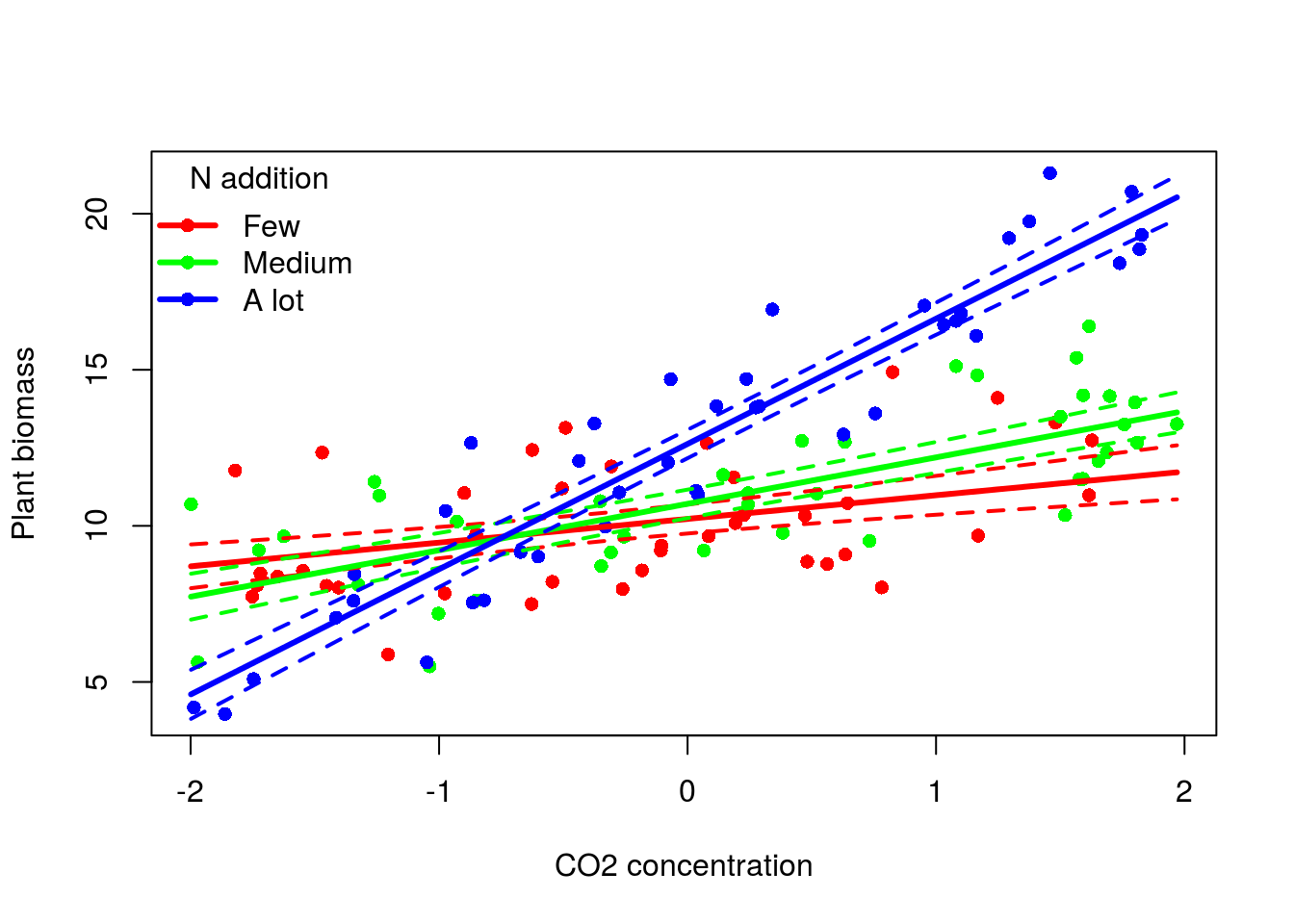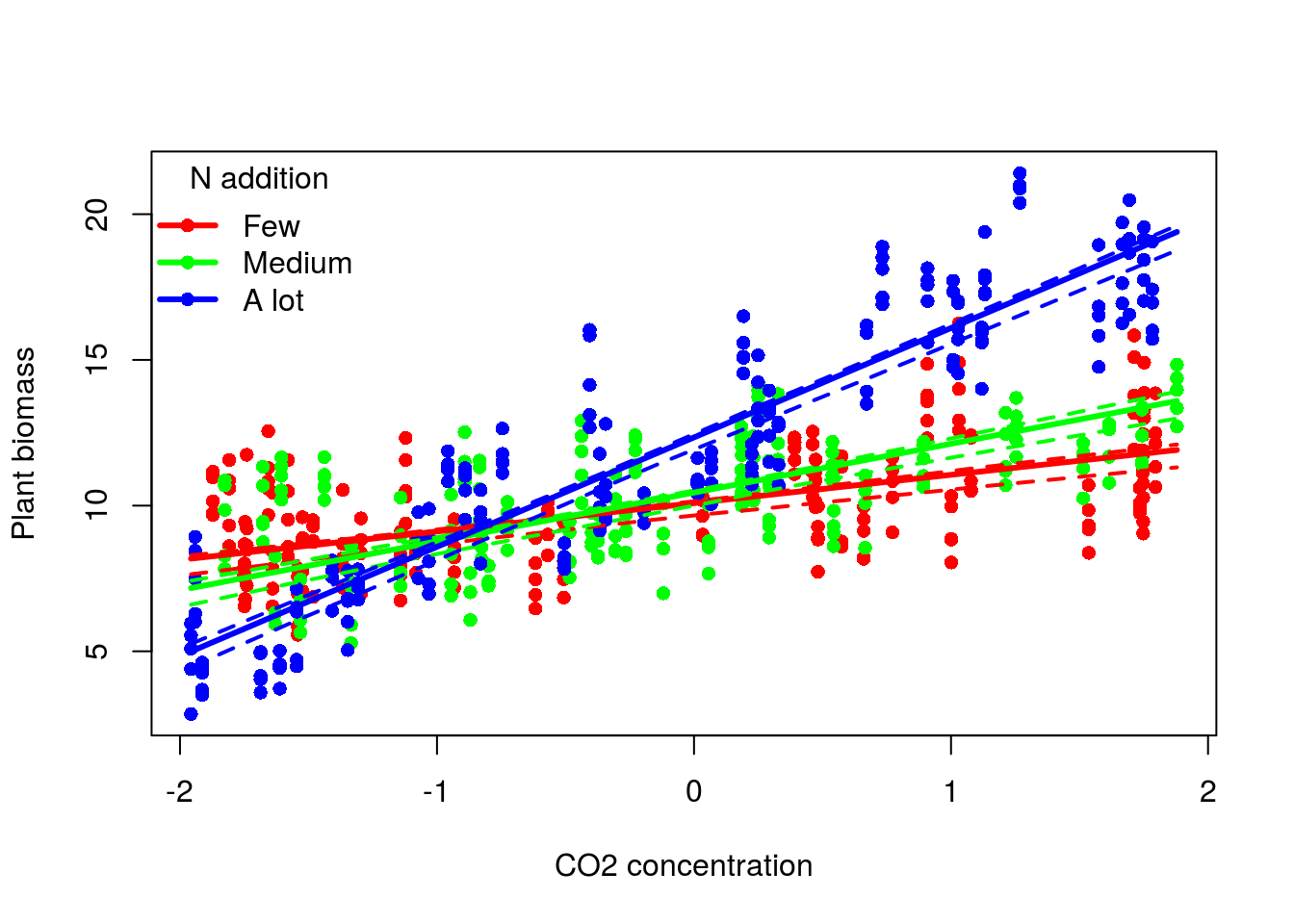Once models have been fitted and checked and re-checked comes the time to interprete them. The easiest way to do so is to plot the response variable versus the explanatory variables (I call them predictors) adding to this plot the fitted regression curve together (if you are feeling fancy) with a confidence interval around it. Now this approach is preferred over the partial residual one because it allows the averaging out of any other potentially confounding predictors and so focus only on the effect of one focal predictor on the response. In my work I have been doing this hundreds of time and finally decided to put all this into a function to clean up my code a little bit. Let’s dive into some code (the function is at the end of the post just copy/paste into your R environment):

#####LM example######
#we measured plant biomass for 120 pots under 3 nutrient treatments and across a gradient of CO2
#due to limited place in our greenhouse chambers we had to use 4 of them, so we established a blocking design
data<-data.frame(C=runif(120,-2,2),N=gl(n = 3,k = 40,labels = c("Few","Medium","A_lot")),Block=rep(rep(paste0("B",1:4),each=10),times=3))
xtabs(~N+Block,data)
##         Block
## N        B1 B2 B3 B4
##   Few    10 10 10 10
##   Medium 10 10 10 10
##   A_lot  10 10 10 10
modmat<-model.matrix(~Block+C*N,data)
#the paramters of the models
params<-c(10,-0.4,2.3,-1.5,1,0.5,2.3,0.6,2.7)
#simulate a response vector
data$Biom<-rnorm(120,modmat%*%params,1) #fit the model m<-lm(Biom~Block+C*N,data) summary(m) ## ## Call: ## lm(formula = Biom ~ Block + C * N, data = data) ## ## Residuals: ## Min 1Q Median 3Q Max ## -2.11758 -0.68801 -0.01582 0.75057 2.55953 ## ## Coefficients: ## Estimate Std. Error t value Pr(>|t|) ## (Intercept) 10.0768 0.2370 42.521 < 2e-16 *** ## BlockB2 -0.3011 0.2690 -1.119 0.265364 ## BlockB3 2.3322 0.2682 8.695 3.54e-14 *** ## BlockB4 -1.4505 0.2688 -5.396 3.91e-07 *** ## C 0.7585 0.1637 4.633 9.89e-06 *** ## NMedium 0.4842 0.2371 2.042 0.043489 * ## NA_lot 2.4011 0.2335 10.285 < 2e-16 *** ## C:NMedium 0.7287 0.2123 3.432 0.000844 *** ## C:NA_lot 3.2536 0.2246 14.489 < 2e-16 *** ## --- ## Signif. codes: 0 '***' 0.001 '**' 0.01 '*' 0.05 '.' 0.1 ' ' 1 ## ## Residual standard error: 1.028 on 111 degrees of freedom ## Multiple R-squared: 0.9201, Adjusted R-squared: 0.9144 ## F-statistic: 159.8 on 8 and 111 DF, p-value: < 2.2e-16 Here we would normally continue and make some model checks. As output from the model we would like to plot the effect of CO2 on plant biomass for each level of N addition. Of course we want to average out the Block effect (otherwise we would have to plot one separate line for each Block). This is how it works: pred<-plot_fit(m,focal_var = "C",inter_var = "N") head(pred) ## C N LC Pred UC ## 1 -1.9984087 Few 8.004927 8.706142 9.407358 ## 2 -1.7895749 Few 8.213104 8.864545 9.515986 ## 3 -1.5807411 Few 8.417943 9.022948 9.627952 ## 4 -1.3719073 Few 8.618617 9.181350 9.744084 ## 5 -1.1630735 Few 8.814119 9.339753 9.865387 ## 6 -0.9542397 Few 9.003286 9.498156 9.993026 #the function output a data frame with columns for the varying predictors #a column for the predicted values (Pred), one lower bound (LC) and an upper one (UC) #let's plot this plot(Biom~C,data,col=c("red","green","blue")[data$N],pch=16,xlab="CO2 concentration",ylab="Plant biomass")
lines(Pred~C,pred[1:20,],col="red",lwd=3)
lines(LC~C,pred[1:20,],col="red",lwd=2,lty=2)
lines(UC~C,pred[1:20,],col="red",lwd=2,lty=2)
lines(Pred~C,pred[21:40,],col="green",lwd=3)
lines(LC~C,pred[21:40,],col="green",lwd=2,lty=2)
lines(UC~C,pred[21:40,],col="green",lwd=2,lty=2)
lines(Pred~C,pred[41:60,],col="blue",lwd=3)
lines(LC~C,pred[41:60,],col="blue",lwd=2,lty=2)
lines(UC~C,pred[41:60,],col="blue",lwd=2,lty=2)
legend("topleft",legend = c("Few","Medium","A lot"),col=c("red","green","blue"),pch=16,lwd=3,title = "N addition",bty="n")The cool thing is that the function will also work for GLM, LMM and GLMM. For mixed effect models the confidence interval is computed from parametric bootstrapping:

#now let's say that we took 5 measurements per pots and we don't want to aggregate them
data<-data.frame(Pots=rep(paste0("P",1:120),each=5),Block=rep(rep(paste0("B",1:4),each=5*10),times=3),N=gl(n = 3,k = 40*5,labels=c("Few","Medium","A_lot")),C=rep(runif(120,-2,2),each=5))
#a random intercept term
rnd_int<-rnorm(120,0,0.4)
modmat<-model.matrix(~Block+C*N,data)
lin_pred<-modmat%*%params+rnd_int[factor(data$Pots)] data$Biom<-rnorm(600,lin_pred,1)
m<-lmer(Biom~Block+C*N+(1|Pots),data)
summary(m)
## Linear mixed model fit by REML ['lmerMod']
## Formula: Biom ~ Block + C * N + (1 | Pots)
##    Data: data
##
## REML criterion at convergence: 1765.1
##
## Scaled residuals:
##     Min      1Q  Median      3Q     Max
## -2.6608 -0.6446 -0.0340  0.6077  3.2002
##
## Random effects:
##  Groups   Name        Variance Std.Dev.
##  Pots     (Intercept) 0.1486   0.3855
##  Residual             0.9639   0.9818
## Number of obs: 600, groups:  Pots, 120
##
## Fixed effects:
##             Estimate Std. Error t value
## (Intercept)  9.93917    0.13225   75.15
## BlockB2     -0.42019    0.15153   -2.77
## BlockB3      2.35993    0.15364   15.36
## BlockB4     -1.36188    0.15111   -9.01
## C            0.97208    0.07099   13.69
## NMedium      0.36236    0.13272    2.73
## NA_lot       2.25624    0.13189   17.11
## C:NMedium    0.70157    0.11815    5.94
## C:NA_lot     2.78150    0.10764   25.84
##
## Correlation of Fixed Effects:
##           (Intr) BlckB2 BlckB3 BlckB4 C      NMedim NA_lot C:NMdm
## BlockB2   -0.572
## BlockB3   -0.575  0.493
## BlockB4   -0.576  0.495  0.493
## C          0.140 -0.012 -0.018 -0.045
## NMedium   -0.511  0.003  0.027  0.007 -0.118
## NA_lot    -0.507  0.008  0.003  0.003 -0.119  0.502
## C:NMedium -0.134  0.019  0.161  0.038 -0.601  0.175  0.071
## C:NA_lot  -0.107  0.077  0.020  0.006 -0.657  0.078  0.129  0.394
#again model check should come here
#for LMM and GLMM we also need to pass as character (vector) the names of the random effect
pred<-plot_fit(m,focal_var = "C",inter_var = "N",RE = "Pots")
#let's plot this
plot(Biom~C,data,col=c("red","green","blue")[data$N],pch=16,xlab="CO2 concentration",ylab="Plant biomass") lines(Pred~C,pred[1:20,],col="red",lwd=3) lines(LC~C,pred[1:20,],col="red",lwd=2,lty=2) lines(UC~C,pred[1:20,],col="red",lwd=2,lty=2) lines(Pred~C,pred[21:40,],col="green",lwd=3) lines(LC~C,pred[21:40,],col="green",lwd=2,lty=2) lines(UC~C,pred[21:40,],col="green",lwd=2,lty=2) lines(Pred~C,pred[41:60,],col="blue",lwd=3) lines(LC~C,pred[41:60,],col="blue",lwd=2,lty=2) lines(UC~C,pred[41:60,],col="blue",lwd=2,lty=2) legend("topleft",legend = c("Few","Medium","A lot"),col=c("red","green","blue"),pch=16,lwd=3,title = "N addition",bty="n")Please note a few elements: - so far the function only return 95% confidence intervals - I have tested it on various types of models that I usually build but there are most certainly still some bugs hanging around so if the function return an error please let me know of the model you fitted and the error returned - the bootstrap computation can take some time for GLMM so be ready to wait a few minute if you have a big complex model - the function accept a vector of variable names for the inter_var argument, it should also work for the RE argument even if I did not tried it yet Happy plotting! Here is the code for the function: #for lm, glm, lmer and glmer models #parameters: #@m : the fitted lm, glm or merMod object (need to be provided) #@focal_var: a character, the name of variable of interest that will be plotted on the x axis, ie the varying variable (need to be provided) #@inter_var: a character or character vector, the name of variable interacting with the focal variable, ie categorical variables from which prediction will be drawn for each level across the focal_var gradient #@RE: if giving a merMod object give as character or character vector of the name of the random effects variable (so far I only tried with one RE) #@n: a numeric, the number of data point that will form the gradient of the focal variable #@n_core: the number of core used to compute the bootstrapped CI for GLMM models plot_fit<-function(m,focal_var,inter_var=NULL,RE=NULL,n=20,n_core=4){ require(arm) dat<-model.frame(m) #turn all character variable to factor dat<-as.data.frame(lapply(dat,function(x){ if(is.character(x)){ as.factor(x) } else{x} })) #make a sequence from the focal variable x1<-list(seq(min(dat[,focal_var]),max(dat[,focal_var]),length=n)) #grab the names and unique values of the interacting variables isInter<-which(names(dat)%in%inter_var) if(length(isInter)==1){ x2<-list(unique(dat[,isInter])) names(x2)<-inter_var } if(length(isInter)>1){ x2<-lapply(dat[,isInter],unique) } if(length(isInter)==0){ x2<-NULL } #all_var<-x1 #add the focal variable to this list all_var<-c(x1,x2) #expand.grid on it names(all_var)<-focal_var all_var<-expand.grid(all_var) #remove varying variables and non-predictors dat_red<-dat[,-c(1,which(names(dat)%in%c(focal_var,inter_var,RE,"X.weights."))),drop=FALSE] if(dim(dat_red)==0){ new_dat<-all_var } else{ fixed<-lapply(dat_red,function(x) if(is.numeric(x)) mean(x) else factor(levels(x),levels = levels(x))) #the number of rows in the new_dat frame fixed<-lapply(fixed,rep,dim(all_var)) #create the new_dat frame starting with the varying focal variable and potential interactions new_dat<-cbind(all_var,as.data.frame(fixed)) #get the name of the variable to average over, debug for conditions where no variables are to be avergaed over name_f<-names(dat_red)[sapply(dat_red,function(x) ifelse(is.factor(x),TRUE,FALSE))] } #get the predicted values cl<-class(m) if(cl=="lm"){ pred<-predict(m,newdata = new_dat,se.fit=TRUE) } if(cl=="glm" | cl=="negbin"){ #predicted values on the link scale pred<-predict(m,newdata=new_dat,type="link",se.fit=TRUE) } if(cl=="glmerMod" | cl=="lmerMod"){ pred<-list(fit=predict(m,newdata=new_dat,type="link",re.form=~0)) #for bootstrapped CI new_dat<-cbind(new_dat,rep(0,dim(new_dat))) names(new_dat)[dim(new_dat)]<-as.character(formula(m)[]) mm<-model.matrix(formula(m,fixed.only=TRUE),new_dat) } #average over potential categorical variables if(length(name_f)>0){ if(cl=="glmerMod" | cl=="lmerMod"){ coef_f<-lapply(name_f,function(x) fixef(m)[grep(paste0("^",x,"\\w+$"),names(fixef(m)))])
}
else{
coef_f<-lapply(name_f,function(x) coef(m)[grep(paste0("^",x,"\\w+$"),names(coef(m)))]) } pred$fit<-pred$fit+sum(unlist(lapply(coef_f,function(x) mean(c(0,x))))) } #to get the back-transform values get the inverse link function linkinv<-family(m)$linkinv

#get the back transformed prediction together with the 95% CI for LM and GLM
if(cl=="glm" | cl=="lm"){
pred$pred<-linkinv(pred$fit)
pred$LC<-linkinv(pred$fit-1.96*pred$se.fit) pred$UC<-linkinv(pred$fit+1.96*pred$se.fit)
}

#for GLMM need to use bootstrapped CI, see ?predict.merMod
if(cl=="glmerMod" | cl=="lmerMod"){
pred$pred<-linkinv(pred$fit)
predFun<-function(.) mm%*%fixef(.)
bb<-bootMer(m,FUN=predFun,nsim=200,parallel="multicore",ncpus=n_core) #do this 200 times
bb$t<-apply(bb$t,1,function(x) linkinv(x))
#as we did this 200 times the 95% CI will be bordered by the 5th and 195th value
bb_se<-apply(bb$t,1,function(x) x[order(x)][c(5,195)]) pred$LC<-bb_se[1,]
pred$UC<-bb_se[2,] } #the output out<-as.data.frame(cbind(new_dat[,1:(length(inter_var)+1)],pred$LC,pred$pred,pred$UC))
names(out)<-c(names(new_dat)[1:(length(inter_var)+1)],"LC","Pred","UC")
return(out)
}